# Key-driver Analysis in PythonThis tutorial walks through doing ‘key driver’ analysis in Python using the proper statistical tools, breaking away from the FiveThirtyEight methodology.

In a Data Science interview a few months ago, I was challenged to use a small data set from our friends at FiveThirtyEight to suggest how best to design a good-selling candy. “Based on ‘market research’ you see here,” the prompt gestured, “advise the product design team on the best features of a candy that we can sell alongside brand-name candies.”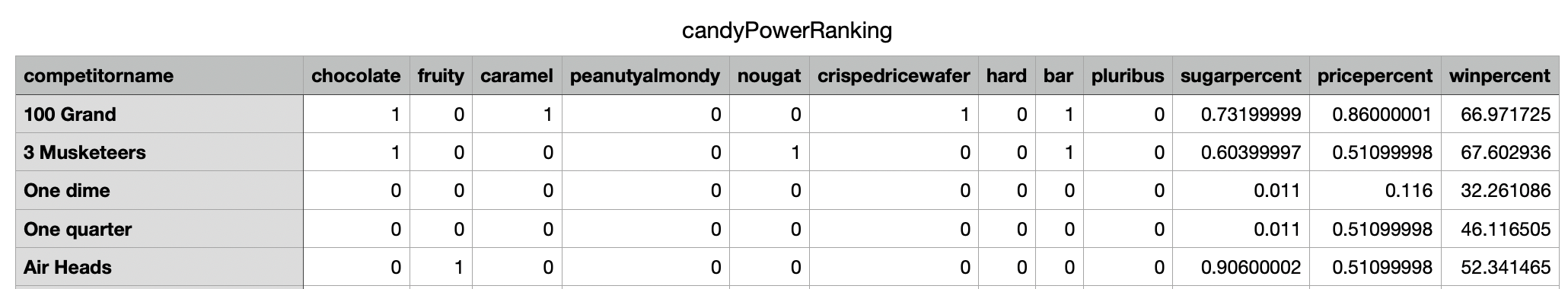The original dataset from FiveThirtyEight, available online here

As a data scientist in the applied, commercial world, the word best _is always a weasel word intended to test your business awareness. One of the tell-tale signs of a greener data scientist is whether they’re thinking about the best business outcome vs. the best machine learning model. ‘Best’ is a balance of ‘what candy elements drive the highest _satisfaction/enjoyment?’ and ‘what candy elements drive the highest price?’ We’re basically trying to find a balance between

1. a candy guaranteed to delight consumers, that
2. occupies a niche enough space such that it’s not just ‘knock-off, discount M&Ms’, and
3. is also cost-optimized to increase profit margins by being cheaper than M&Ms.

Our friends at FiveThirtyEight made a grave statistical error (or two) when trying to solve for #1.

This tutorial walks through doing ‘key driver’ analysis in python using the proper statistical tools, breaking away from the FiveThirtyEight methodology. Along the way, I explain 1) why data scientists and product strategists should trust my numbers more, and 2) how to communicate those results in a way that gains that trust (see my candy dashboard).

• Methodology: linear regression isn’t right, use relative weight analysis instead
• Implementation: Doing RWA in python for candy flavor and price
• Triangulation: Why the business should trust the RWA via triangulation

## Statistics First, ML Second

Let’s set out for our statistical methodology by way of understanding why linear regression is not the right answer… or at least not _really _the right answer.

FiveThirtyEight builds a multiple regression, including all possible features of a candy captured in their data set. Importance is abstracted from the coefficients of the linear regression, using the P-value of the dimension to define whether we can take it as reliable.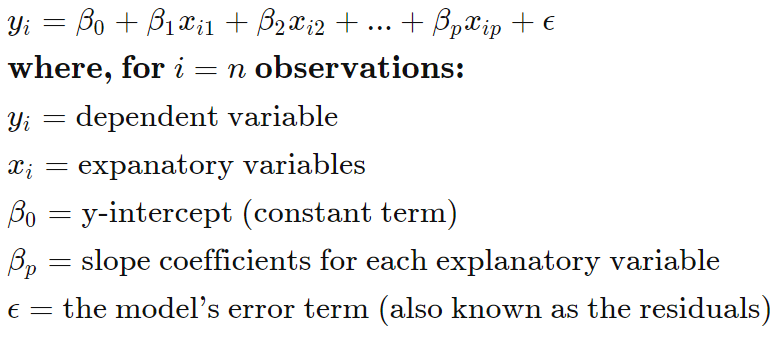Equation for multiple regression

However, looking at the equation for a linear regression, we see a pretty significant problem. Remember: an OLS regression coefficient tells us whether an increase in the independent variable correlates to an increase in the mean of the dependent variable (and vice versa for negative). But it’s measure of magnitude.

In our candy problem, if we build an OLS regression to predict the winning-ness of a candy-bar, and we change the units from grams to pounds, we would get a much higher coefficient. The volume didn’t change anything besides units. The argument here might be made that you can standardize your variables, such as normalizing to 0 mean and unit variance. Still yet, with normalization, you may have an issue of collinearity — if predictors are linearly dependent or highly correlated, the OLS becomes unstable, which will be true even when the independent variables are standardized.

So we need another tool in our toolbelt. Something that can help us get around the bad assumptions about coefficients. While ML may not have the answer, statistics does.

## Relative Weight Analysis and why R2 is the real jedi

Here, we’ll implement that will tell us how much each feature/independent variable contributes to criterion variance (R2). In its raw form, Relative Weight Analysis returns raw importance scores whose sum equals to the overall R2 of a model; it’s normalized form allows us to say “Feature X _accounts for _Z% _of variance in target variable _Y.” Or, more concretely,

“Assuming that a key driver of what makes a candy popular is captured here, Chocolates with nuts is the winning-est flavor combination.”

Relative weight analysis relies on the decomposition of R2 to assign importance to each predictor. Where intercorrelations between independent variables make it near impossible to take standardized regression weights as measure of importance, RWA solves this problem by creating predictors that are orthogonal to one another and regressing on these without the effects of multicollinearity. They are then transformed back to the metric of the original predictors.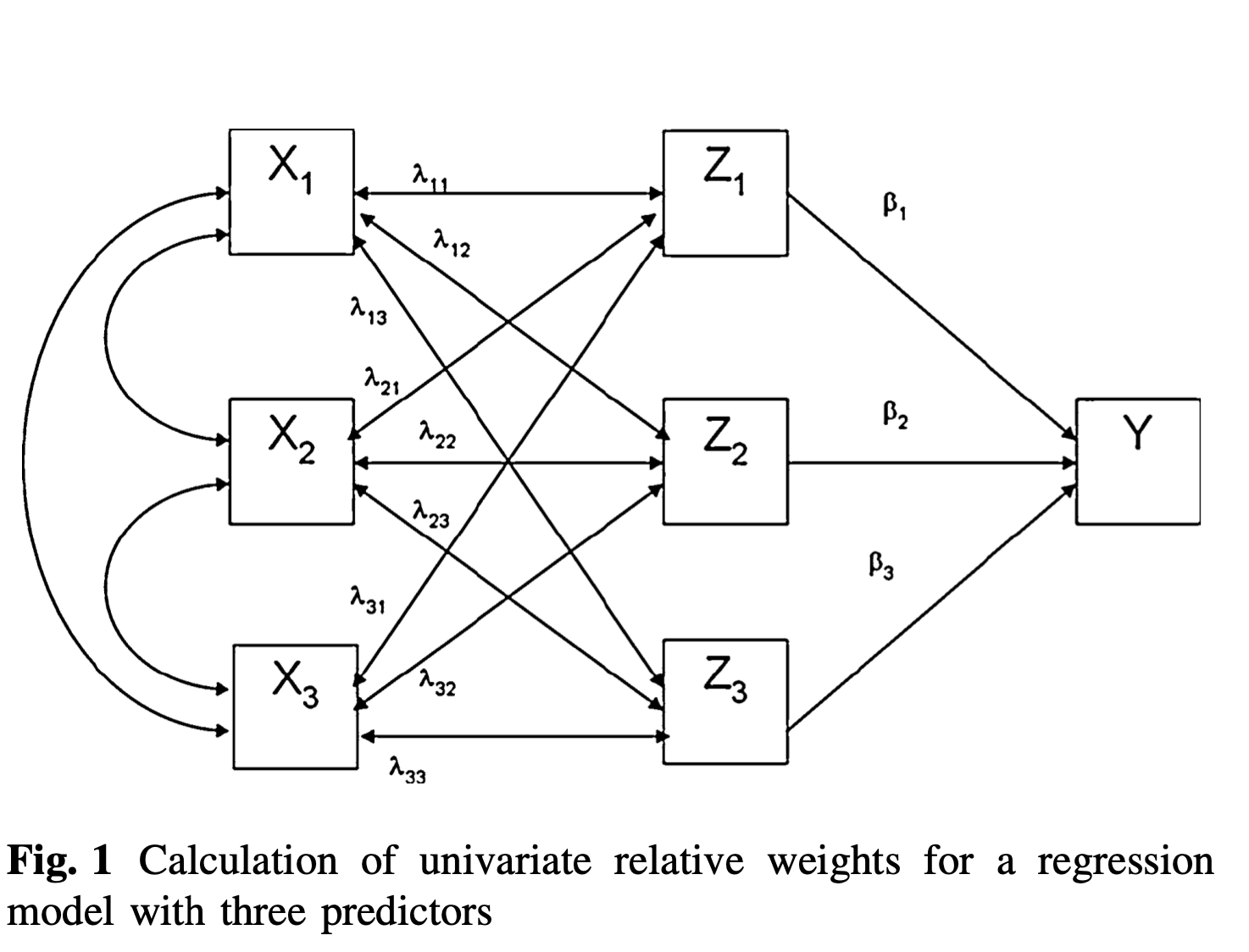Example of 3-feature RWA, from here

We have multiple steps involved, and rather than demonstrate in notation, I’ll walk through a python script that you’ll be able to use (please credit this post if you use this verbatim or spin-off your own version!) I’m assuming that at this point you’ve done your EDA and manipulations necessary to build a logically and mathematically sound model.

## Implementing RWA in Python

Step 1: Get a correlation between all of the dependent and independent variables.

``````corr_matrix = df[feature_names].apply(pd.to_numeric, errors = ‘coerce’).corr()
corr_X = corr_matrix.iloc[1:, 1:].copy()
corr_Xy = corr_matrix.iloc[1:, 0].copy()``````

Step 2: Create orthogonal predictors using eigenvectors and eigenvalues on the correlation matrix, creating a diagonal matrix of the square root of eigenvalues. This gets around the issue of multi-collinearity. Note the python tricks for getting the diagonal indices.

``````w_corr_Xs, v_corr_Xs = np.linalg.eig(corr_Xs)
diag_idx = np.diag_indices(len(corr_Xs)
diag = np.zeros((num_drivers, num_drivers), float)
diag[diag_idx] = w_corr_Xs
delta = np.sqrt(diag)``````

Step 3: Multiply the eigenvector matrix and its transposition (in python, we can use @ as an operator, called matmul). This allows us to treat X as the set of dependent variables, regressing X onto matrix Z — itself the orthogonal counterpart of X having the least squared error. To get the partial effect of each independent variable, we apply matrix multiplication to the inverse and correlation matricies.

``````coef_xz = v_corr_Xs @ delta @ v_corr_Xs.transpose()
coef_yz = np.linalg.inv(coef_xz) @ corr_Xy
``````

NOTE: As mentioned, the sum of the squares of coef_yz above should add up to the total R2! This will be important in the next step!

Step 4: We then calculate the relative weight as the multiplication of the matrix in Step 2 and step 3. The normalized version is then the percentage of r2 that these account for!

``````r2 = sum(np.square(coef_yz))
raw_relative_weights = np.square(coef_xz) @ np.square(coef_yz)
normalized_relative_weights = (raw_relative_weights/r2)*100``````

Now, you can just zip up your features and these two lists to get the relative weight of each one as it ‘drives’ (or, more mathematically, accounts for variance in relation to increases in) the percentage of wins in the candy duals.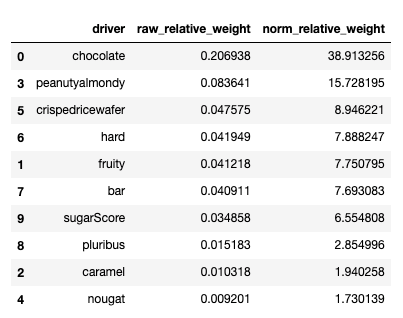Raw & Normal relative weights for predictiveness of winning-ness

Chocolate and nuts wins flavors, but we’re not done. We have to also make money by generating value from the candy we create, measured by revenue minus costs. Using price percentiles in our table, we can also look at what drives the prices of candy.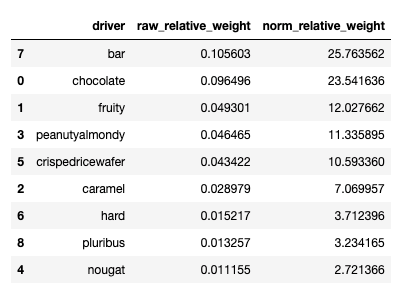Raw & Normal relative weights for predictiveness of price percentile

## Hire Machine Learning Developers in India

We supply you with world class machine learning experts / ML Developers with years of domain experience who can add more value to your business.

## Applied Data Analysis in Python Machine Learning and Data Science | Scikit-Learn

Applied Data Analysis in Python Machine learning and Data science, we will investigate the use of scikit-learn for machine learning to discover things about whatever data may come across your desk.

## Hire Machine Learning Developer | Hire ML Experts in India

We supply you with world class machine learning experts / ML Developers with years of domain experience who can add more value to your business.

## Data Science Projects | Data Science | Machine Learning | Python

Practice your skills in Data Science with Python, by learning and then trying all these hands-on, interactive projects, that I have posted for you.

## Data Science Projects | Data Science | Machine Learning | Python

Practice your skills in Data Science with Python, by learning and then trying all these hands-on, interactive projects, that I have posted for you.